As part of the “Tutorial on Graph Neural Networks for Computer Vision and Beyond”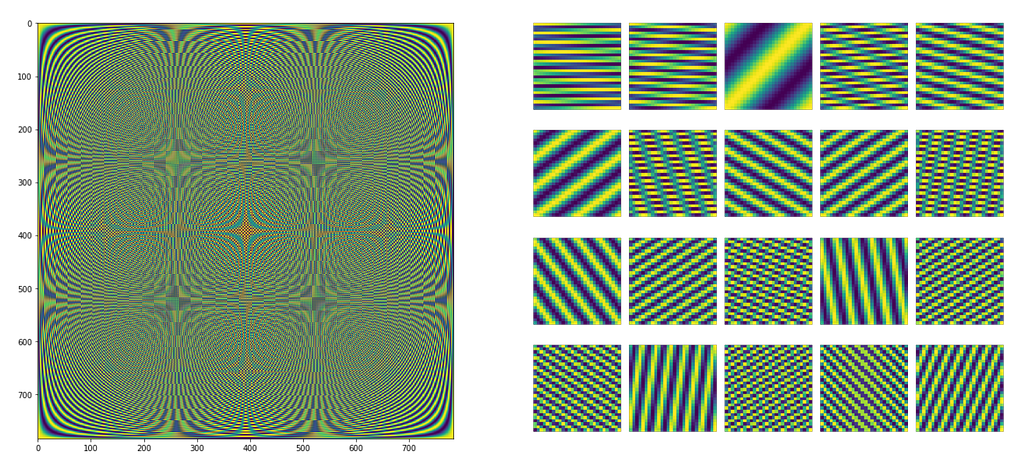The Fourier basis (DFT matrix) on the left, in which each column or row is a basis vector (on the right). The Fourier basis is used to compute spectral convolution is signal processing. In graphs, the Laplacian basis is used described in this post.

First, let’s recall what is a graph. A graph G is a set of nodes (vertices) connected by directed/undirected edges. In this post, I will assume an undirected graph G with N nodes. Each node in this graph has a C-dimensional feature vector, and features of all nodes are represented as an N×C dimensional matrix X⁽ˡ⁾. Edges of a graph are represented as an N×N matrix A, where the entry Aᵢⱼ indicates if node i is connected (adjacent) to node j. This matrix is called an adjacency matrix.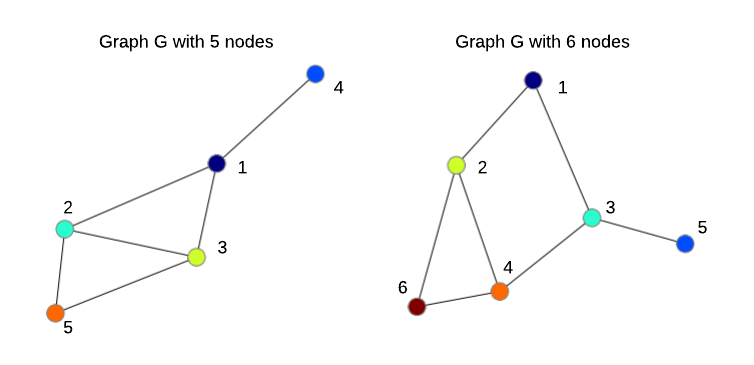Two undirected graphs with N=5 and N=6 nodes. The order of nodes is arbitrary.

Spectral analysis of graphs (see lecture notes here and earlier work here) has been useful for graph clustering, community discovery and other mainly unsupervised learning tasks. In this post, I basically describe the work of Bruna et al., 2014, ICLR 2014 who combined spectral analysis with convolutional neural networks (ConvNets) giving rise to spectral graph convolutional networks that can be trained in a supervised way, for example for the graph classification task.

Despite that spectral graph convolution is currently less commonly used compared to spatial graph convolution methods, knowing how spectral convolution works is still helpful to understand and avoid potential problems with other methods. Plus, in the conclusion I refer to some recent exciting works making spectral graph convolution more competitive.

1. Graph Laplacian and a little bit of physics

While “spectral” may sound complicated, for our purpose it’s enough to understand that it simply means decomposing a signal/audio/image/graph into a combination (usually, a sum) of simple elements (wavelets, graphlets). To have some nice properties of such a decomposition, these simple elements are usually orthogonal, i.e. mutually linearly independent, and therefore form a basis.

When we talk about “spectral” in signal/image processing, we imply the Fourier Transform, which offers us a particular basis (DFT matrix, e.g. scipy.linalg.dft in Python) of elementary sine and cosine waves of different frequencies, so that we can represent our signal/image as a sum of these waves. But when we talk about graphs and graph neural networks (GNNs), “spectral” implies eigen-decomposition of the graph Laplacian L. You can think of the the graph Laplacian L as an adjacency matrix A normalized in a special way, whereas eigen-decomposition is a way to find those elementary orthogonal components that make up our graph.

Intuitively, the graph Laplacian shows in what directions and how smoothly the “energy” will diffuse over a graph if we put some “potential” in node i. A typical use-case of Laplacian in mathematics and physics is to solve how a signal (wave) propagates in a dynamic system. Diffusion is smooth when there is no sudden changes of values between neighbors as in the animation below.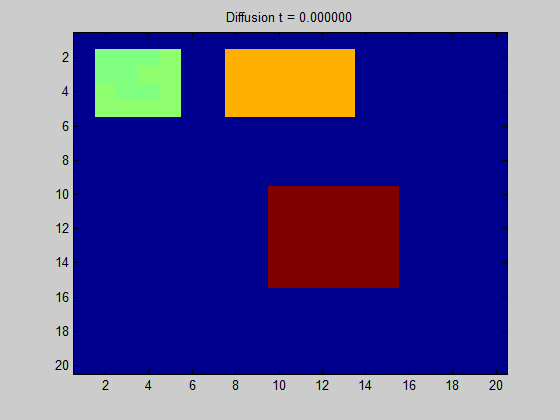Diffusion of some signal (for example, it can be heat) in a regular grid graph computed based on the graph Laplacian (source). Basically, the only things required to compute these dynamics are the Laplacian and initial values in nodes (pixels), i.e. red and yellow pixels corresponding to high intensity (of heat).

In the rest of the post, I’m going to assume “symmetric normalized Laplacian”, which is often used in graph neural networks, because it is normalized so that when you stack many graph layers, the node features propagate in a more smooth way without explosion or vanishing of feature values or gradients. It is computed based only on an adjacency matrix A of a graph, which can be done in a few lines of Python code as follows:

# Computing the graph Laplacian
# A is an adjacency matrix of some graph G
import numpy as np
N = A.shape # number of nodes in a graph
D = np.sum(A, 0) # node degrees
D_hat = np.diag((D + 1e-5)**(-0.5)) # normalized node degrees
L = np.identity(N) — np.dot(D_hat, A).dot(D_hat) # Laplacian

Here, we assume that A is symmetric, i.e. A = Aᵀ and our graph is undirected, otherwise node degrees are not well-defined and some assumptions must be made to compute the Laplacian. An interesting property of an adjacency matrix A is that Aⁿ (matrix product taken n times) exposes n-hop connections between nodes (see here for more details).

Let’s generate three graphs and visualize their adjacency matrices and Laplacians as well as their powers.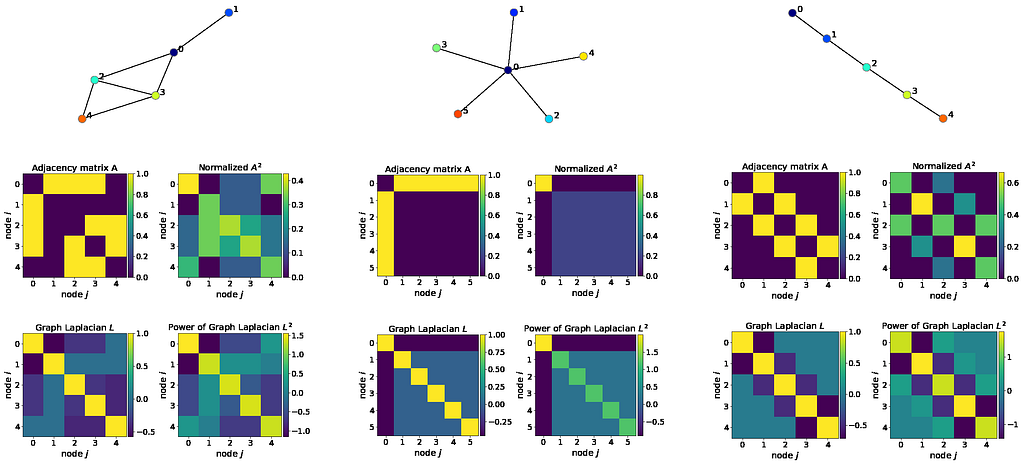Adjacency matrices, Laplacians and their powers for a random graph (left), “star graph” (middle) and “path graph” (right). I normalize A² such that the sum in each row equals 1 to have a probabilistic interpretation of 2-hop connections. Notice that Laplacians and their powers are symmetric matrices, which makes eigen-decomposition easier as well as facilitates feature propagation in a deep graph network.

For example, imagine that the star graph above in the middle is made from metal, so that it transfers heat well. Then, if we start to heat up node 0 (dark blue), this heat will propagate to other nodes in a way defined by the Laplacian. In the particular case of a star graph with all edges equal, heat will spread uniformly to all other nodes, which is not true for other graphs due to their structure.

In the context of computer vision and machine learning, the graph Laplacian defines how node features will be updated if we stack several graph neural layers. Similarly to the first part of my tutorial, to understand spectral graph convolution from the computer vision perspective, I’m going to use the MNIST dataset, which defines images on a 28×28 regular grid graph.MNIST image defining features X (left), adjacency matrix A (middle) and the Laplacian (right) of a regular 28×28 grid. The reason that the graph Laplacian looks like an identity matrix is that the graph has a relatively large number of nodes (784), so that after normalization values outside the diagonal become much smaller than 1.

2. Convolution

In signal processing, it can be shown that convolution in the spatial domain is multiplication in the frequency domain (a.k.a. convolution theorem). The same theorem can be applied to graphs. In signal processing, to transform a signal to the frequency domain, we use the Discrete Fourier Transform, which is basically matrix multiplication of a signal with a special matrix (basis, DFT matrix). This basis assumes a regular grid, so we cannot use it for irregular graphs, which is a typical case. Instead, we use a more general basis, which is eigenvectors V of the graph Laplacian L, which can be found by eigen-decomposition: L=VΛVᵀ, where Λ are eigenvalues of L.

PCA vs eigen-decomposition of the graph Laplacian. To compute spectral graph convolution in practice, it’s enough to use a few eigenvectors corresponding to the smallest eigenvalues. At first glance, it seems to be an opposite strategy compared to frequently used in computer vision Principal component analysis (PCA), where we are more interested in the eigenvectors corresponding to the largest eigenvalues. However, this difference is simply due to the negation used to compute the Laplacian above, therefore eigenvalues computed using PCA are inversely proportional to eigenvalues of the graph Laplcacian (see this paper for a formal analysis). Note also that PCA is applied to the covariance matrix of a dataset for the purpose to extract the largest factors of variation, i.e. the dimensions along which data vary the most, like in Eigenfaces. This variation is measured by eigenvalues, so that the smallest eigenvalues essentially correspond to noisy or “spurious” features, which are assumed to be useless or even harmful in practice.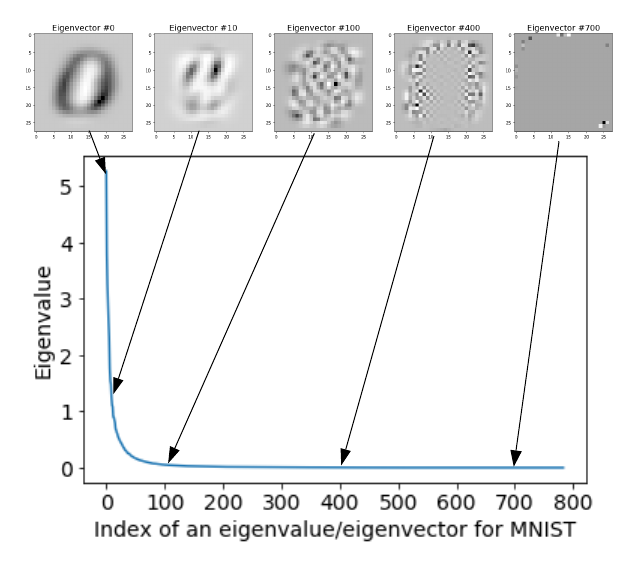Eigenvalues (in a descending order) and corresponding eigenvectors for the MNIST dataset.

Eigen-decomposition of the graph Laplacian is applied to a single graph for the purpose to extract subgraphs or clusters (communities) of nodes, and eigenvalues tell us a lot about graph connectivity. I will use eigenvectors corresponding to the 20 smallest eigenvalues in our examples below, assuming that 20 is much smaller than the number of nodes N (N=784 in case of MNIST). To find eigenvalues and eigenvectors below on the left, I use a 28×28 regular graph, whereas on the right I follow the experiment of Bruna et al. and construct an irregular graph by sampling 400 random locations on a 28×28 regular grid (see their paper for more details about this experiment).Eigenvalues Λ (bottom) and eigenvectors V (top) of the graph Laplacian L for a regular 28×28 grid (left) and non-uniformly subsampled grid with 400 points according to experiments in Bruna et al., 2014, ICLR 2014 (right). Eigenvectors corresponding to the 20 smallest eigenvalues are shown. Eigenvectors are 784 dimensional on the left and 400 dimensional on the right, so V is 784×20 and 400×20 respectively. Each of the 20 eigenvectors on the left was reshaped to 28×28, whereas on the right to reshape a 400 dimensional eigenvector to 28×28, white pixels for missing nodes were added. So, each pixel in each eigenvector corresponds to a node or a missing node (in white on the right). These eigenvectors can be viewed as a basis in which we decompose our graph.

So, given graph Laplacian L, node features X and filters W_spectral, in Python spectral convolution on graphs looks very simple:

# Spectral convolution on graphs
# X is an N×1 matrix of 1-dimensional node features
# L is an N×N graph Laplacian computed above
# W_spectral are
F weights (filters) that we want to train
from scipy.sparse.linalg import eigsh # assumes L to be symmetric
Λ,V = eigsh(L,k=20,which=’SM’) # eigen-decomposition (i.e. find Λ,V)
X_hat = V.T.dot(X) # 20×1 node features in the "spectral" domain
W_hat = V.T.dot(W_spectral) # 20×F filters in the "spectral" domain
Y = V.dot(X_hat * W_hat) # F result of convolution

Formally:Spectral graph convolution, where ⊙ means element-wise multiplication.

where we assume that our node features X⁽ˡ⁾ are 1-dimensional, e.g. MNIST pixels, but it can be extended to a C-dimensional case: we will just need to repeat this convolution for each channel and then sum over C as in signal/image convolution.

Formula (3) is essentially the same as spectral convolution of signals on regular grids using the Fourier Transform, and so creates a few problems for machine learning:

• the dimensionality of trainable weights (filters) W_spectral depends on the number of nodes N in a graph;
• W_spectral also depends on the graph structure encoded in eigenvectors V.

These issues prevent scaling to datasets with large graphs of variable structure. Further efforts, summarized below, were focused on resolving these and other issues.

3. “Smoothing” in the spectral domainStrawberry and banana smoothie (source: joyfoodsunshine.com). Smoothing in the spectral domain is a little bit different 😃.

Bruna et al. were one of the first to apply spectral graph analysis to learn convolutional filters for the graph classification problem. The filters learned using formula (3) above act on the entire graph, i.e. they have global support. In the computer vision context, this would be the same as training convolutional filters of size 28×28 pixels on MNIST, i.e. filters have the same size as the input (note that we would still slide a filter, but over a zero-padded image). While for MNIST we can actually train such filters, the common wisdom suggests to avoid that, as it makes training much harder due to the potential explosion of the number of parameters and difficulty of training large filters that can capture useful features shared across different images.

I actually successfully trained such a model using PyTorch and this code from my GitHub. You should run it using mnist_fc.py --model conv. After training for 100 epochs, the filters look like mixtures of digits: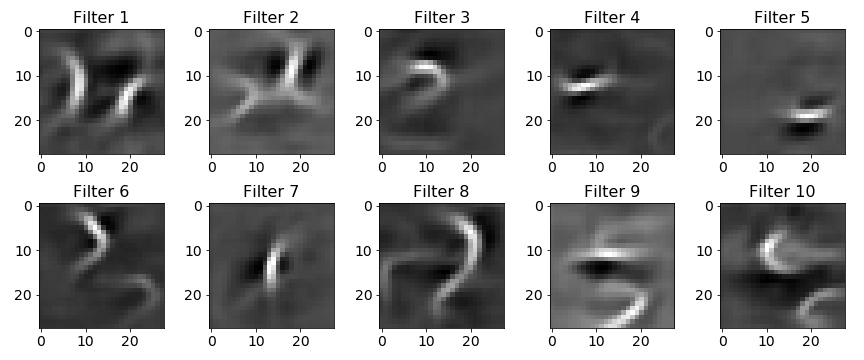Examples of filters with global support typically used in spectral convolution. In this case, these are 28×28 filters learned using a ConvNet with a single convolutional layer followed by ReLU, 7×7 MaxPooling and a fully-connected classification layer. To make it clear, the output of the convolutional layer is still 28×28 due to zero-padding. Surprisingly, this net achieves 96.7% on MNIST. This can be explained by the simplicity of the dataset.

To reiterate, we generally want to make filters smaller and more local (which is not exactly the same as I’ll note below).

To enforce that implicitly, they proposed to smooth filters in the spectral domain, which makes them more local in the spatial domain according to the spectral theory. The idea is that you can represent our filter W_spectral from formula (3) as a sum of 𝐾 predefined functions, such as splines, and instead of learning N values of W, we learn K coefficients α of this sum:We can approximate our N dimensional filter W_spectral as a finite sum of K functions f, such as splines shown below. So, instead of learning N values of W_spectral, we can learn K coefficients (alpha) of those functions; it becomes efficient when K << N.

While the dimensionality of fk does depend on the number of nodes N, these functions are fixed, so we don’t learn them. The only thing we learn are coefficients α, and so W_spectral is no longer dependent on N. Neat, right?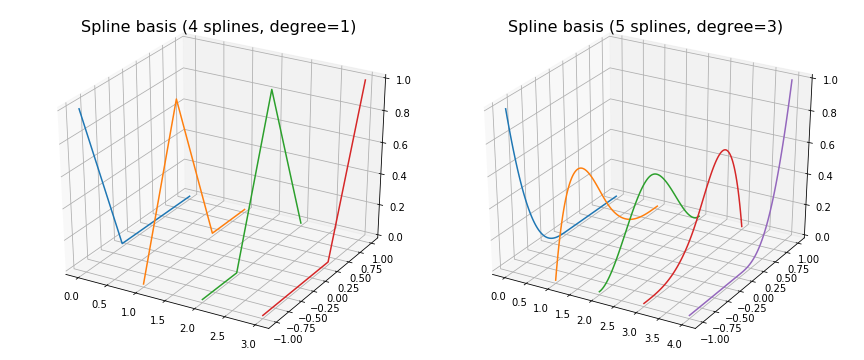The spline basis used to smooth filters in the frequency domain, thereby making them more local. Splines and other polynomial functions are useful, because we can represent filters as their sums.

To make our approximation in formula (4) reasonable, we want K<<N to reduce the number of trainable parameters from N to K and, more importantly, make it independent of N, so that our GNN can digest graphs of any size. We can use different bases to perform this “expansion”, depending on which properties we need. For instance, cubic splines shown above are known as very smooth functions (i.e. you cannot see knots, i.e. where the pieces of the piecewise spline polynomial meet, except if you use degree 1). The Chebyshev polynomial, which I’ll discuss below, has the minimum 𝑙∞ distance between the approximating function. The Fourier basis is the one that preserves most of the signal energy after transformation. Most bases are orthogonal, because it would be redundant to have terms that can be expressed by each other.

Note that filters W_spectral are still as large as the input, but their effective width is small. In case of MNIST images, we would have 28×28 filters, in which only a small fraction of values would have an absolute magnitude larger than 0 and all of them should be located close to each other, i.e. the filter would be local and effectively small, something like the one below (second from the left):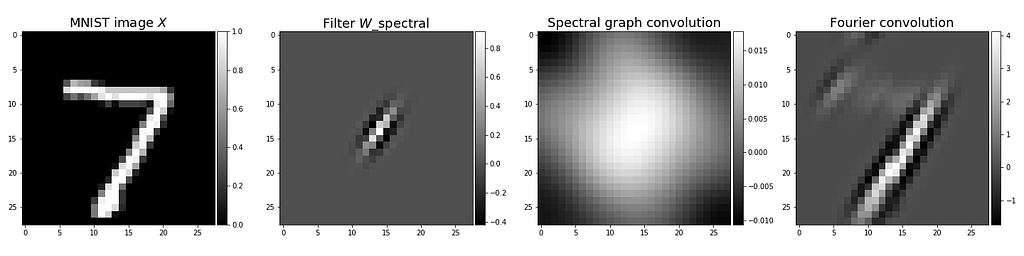From left to right: (first) Input image. (second) Local filter with small effective width. Most values are very close to 0. (third) The result of spectral graph convolution of the MNIST image of digit 7 and the filter. (fourth) The result of spectral convolution using the Fourier transform. These results indicate that spectral graph convolution is quite limited if applied to images, perhaps, due to the weak spatial structure of the Laplacian basis compared to the Fourier basis.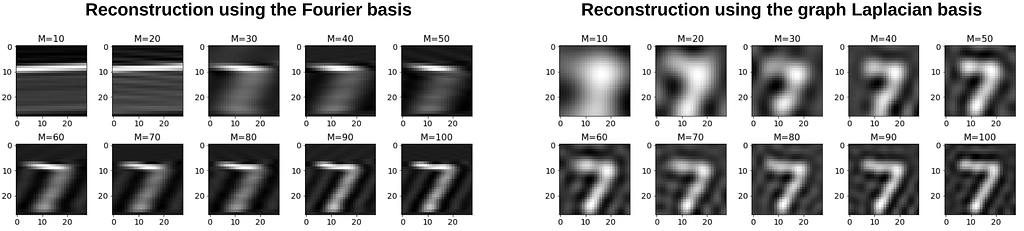Reconstruction of the MNIST image using the Fourier and graph Laplacian bases using only M components of V: X’=V VᵀX. We can see that the bases compress different patterns in images (orientated edges in the Fourier case and global patterns in the Laplacian case). This makes results of convolutions illustrated above very different.

To summarize, smoothing in the spectral domain allowed Bruna et al. to learn more local filters. The model with such filters can achieve similar results as the model without smoothing (i.e. using our formula (3)), but with much fewer trainable parameters, because the filter size is independent of the input graph size, which is important to scale the model to datasets with larger graphs. However, learned filters W_spectral still depend on eigenvectors V, which makes it challenging to apply this model to datasets with variable graph structures.

Conclusion

Despite the drawbacks of the original spectral graph convolution method, it has been developed a lot and has remained a quite competitive method in some applications, because spectral filters can better capture global complex patterns in graphs, which local methods like GCN (Kipf & Welling, ICLR, 2017) cannot unless stacked in a deep network. For example, two ICLR 2019 papers, of Liao et al. on “LanczosNet” and Xu et al. on “Graph Wavelet Neural Network”, address some shortcomings of spectral graph convolution and show great results in predicting molecule properties and node classification. Another interesting work of Levie et al., 2018 on “CayleyNets” showed strong performance in node classification, matrix completion (recommender systems) and community detection. So, depending on your application and infrastructure, spectral graph convolution can be a good choice.

In another part of my Tutorial on Graph Neural Networks for Computer Vision and Beyond I explain Chebyshev spectral graph convolution introduced by Defferrard et al. in 2016, which is still a very strong baseline that has some nice properties and is easy to implement as I demonstrate using PyTorch.

Acknowledgement: A large portion of this tutorial was prepared during my internship at SRI International under the supervision of Mohamed Amer (homepage) and my PhD advisor Graham Taylor (homepage). I also thank Carolyn Augusta for useful feedback.

Find me on Github, LinkedIn and Twitter.Spectral Graph Convolution Explained and Implemented Step By Step was originally published in Towards Data Science on Medium, where people are continuing the conversation by highlighting and responding to this story.